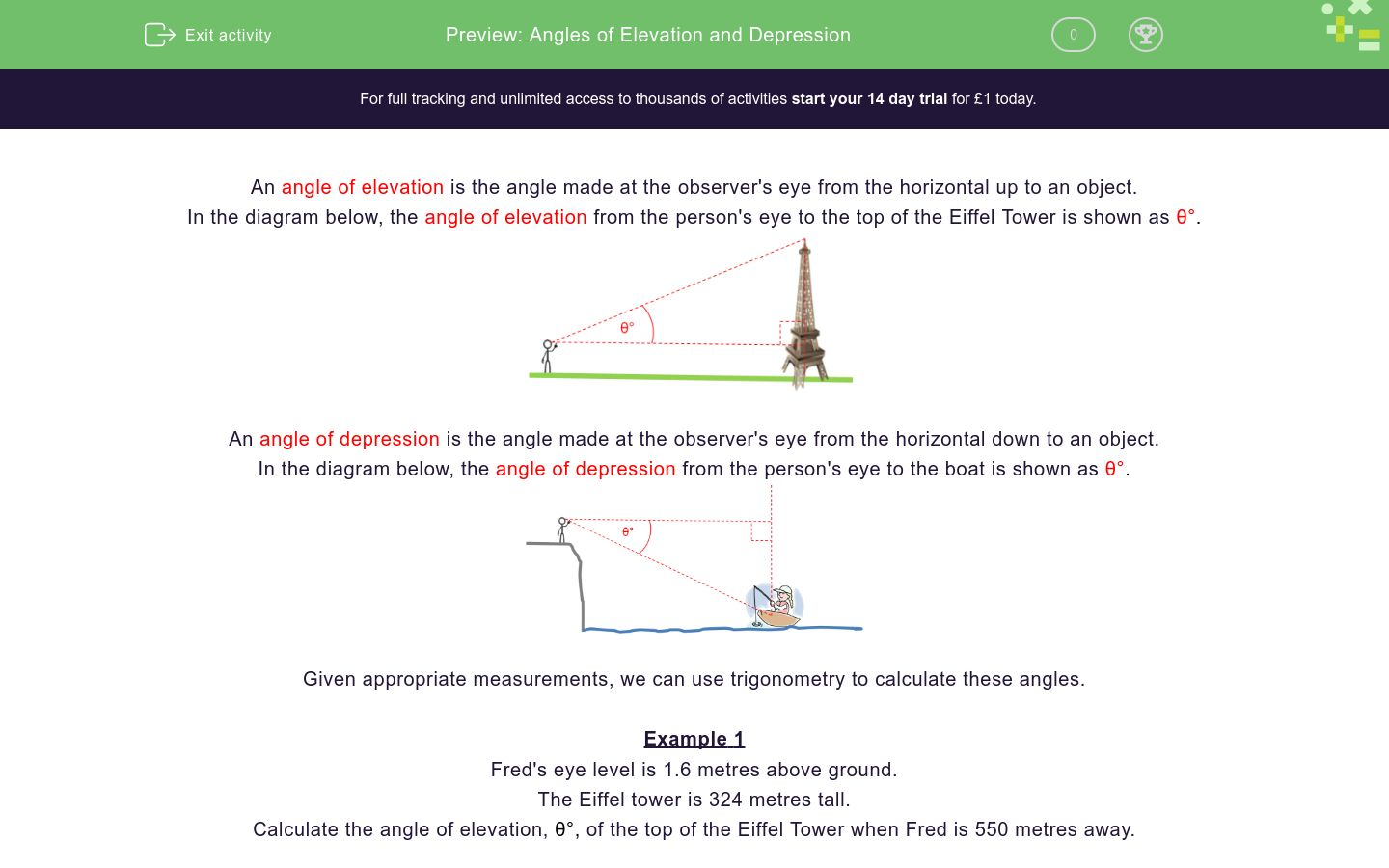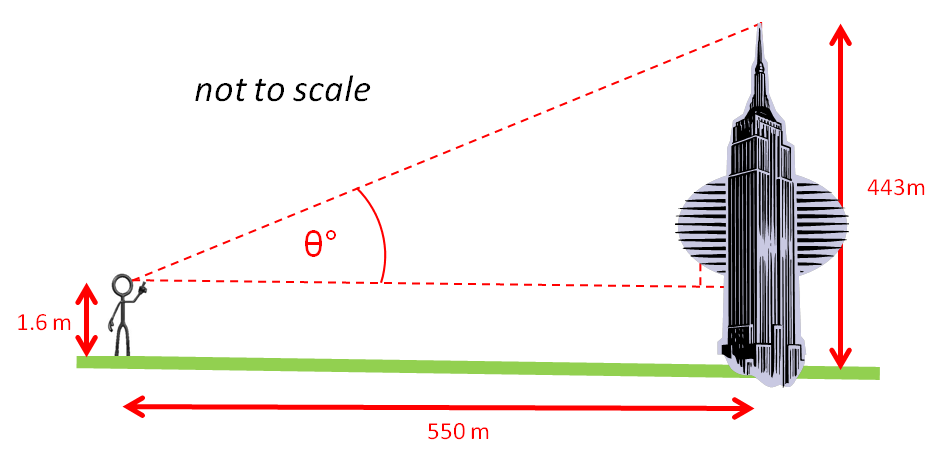# Angles of Elevation and Depression

In this worksheet, students must use trigonometry to answer questions on angles of elevation and depression.Key stage:  KS 4

Curriculum topic:  Geometry and Measures

Difficulty level:### QUESTION 1 of 10

An angle of elevation is the angle made at the observer's eye from the horizontal up to an object.

In the diagram below, the angle of elevation from the person's eye to the top of the Eiffel Tower is shown as θ°.An angle of depression is the angle made at the observer's eye from the horizontal down to an object.

In the diagram below, the angle of depression from the person's eye to the boat is shown as θ°.Given appropriate measurements, we can use trigonometry to calculate these angles.

Example 1

Fred's eye level is 1.6 metres above ground.

The Eiffel tower is 324 metres tall.

Calculate the angle of elevation, θ°, of the top of the Eiffel Tower when Fred is 550 metres away.In relation to the angle shown as θ°, the opposite side of the right-angled triangle has length 324 - 1.6 = 322.4 m and the adjacent side is 550 m.

Thus, tan θ° = 322.4 ÷ 550 = 0.58618...

θ° = tan-1(0.58618) = 30.4°

Example 2

Fred's eye level is 1.6 metres above ground.

He is standing on cliff which is 30 metres above sea level and measures the angle of depression to a boat as 31°.

Calculate the horizontal distance, d,  from Fred to the boat.In relation to the 31° angle of depression, the opposite side of the right-angled triangle has length 30 + 1.6 = 31.6 m and the adjacent side is d m.

Thus, tan 31° = 31.6 ÷ d

d = 31.6 ÷ tan 31° = 52.6 m

Fred's eye level is 1.6 metres above ground.

The Empire State Building is 443 metres tall.

Calculate the angle of elevation, θ°, of the top of the Empire State Building when Fred is 550 metres away.Fred's eye level is 1.6 metres above ground.

He is standing on cliff which is 30 metres above sea level and measures the angle of depression to a boat as 25°.

Calculate the horizontal distance, d,  in metres from Fred to the boat.Fred's eye level is 1.6 metres above ground.

Fred measures the angle of elevation of the top of the skyscraper at 29° when he is 500 metres away.

How tall is the skyscraper in metres?Fred's eye level is 1.6 metres above ground.

He is standing on cliff which is h metres above sea level and measures the angle of depression to a boat 400 m away as 26°.

Calculate the height of the cliff, h, in metres.Fred's eye level is 1.6 metres above ground.

Fred measures the angle of elevation of the top of a 300m high skyscraper at 30°.

How far away from the skyscraper is Fred in metres?Fred's eye level is 1.6 metres above ground.

He is standing on cliff which is 45 metres above sea level and measures the angle of depression to a boat 400 m away.

Calculate the angle of depression, θ°.The diagram below shows a Christmas tree with base at A and top at T.The distance AC is 40 metres.

The angle of elevation of T from C is 32°.

Calculate the height of the tree, AT in metres.

The diagram below shows a Christmas tree with base at A and top at T.The distance AC is 40 metres.

The angle of elevation of T from C is 32°.

The angle of elevation of T from B is 19°.

Calculate the distance, AB in metres.

The diagram below shows a Christmas tree with base at A and top at T.The distance AC is 40 metres.

The angle of elevation of T from C is 32°.

The angle of elevation of T from B is 19°.

Calculate the distance, BC in metres.

The diagram below shows a Christmas tree with base at A and top at T.The distance AC is 28 metres.

The angle of elevation of T from C is 35°.

The angle of elevation of T from B is 12°.

Calculate the distance, BC in metres.

• Question 1

Fred's eye level is 1.6 metres above ground.

The Empire State Building is 443 metres tall.

Calculate the angle of elevation, θ°, of the top of the Empire State Building when Fred is 550 metres away.38.7
EDDIE SAYS
θ° = tan-1((443 - 1.6) ÷ 550)) = 38.7486
• Question 2

Fred's eye level is 1.6 metres above ground.

He is standing on cliff which is 30 metres above sea level and measures the angle of depression to a boat as 25°.

Calculate the horizontal distance, d,  in metres from Fred to the boat.67.8
EDDIE SAYS
d = 31.6 ÷ tan25° = 67.7664...
• Question 3

Fred's eye level is 1.6 metres above ground.

Fred measures the angle of elevation of the top of the skyscraper at 29° when he is 500 metres away.

How tall is the skyscraper in metres?278.8
EDDIE SAYS
(h - 1.6)/500 = tan29°
h - 1.6 = 500tan29° = 277.1545...
h = 278.7545...
• Question 4

Fred's eye level is 1.6 metres above ground.

He is standing on cliff which is h metres above sea level and measures the angle of depression to a boat 400 m away as 26°.

Calculate the height of the cliff, h, in metres.193.5
EDDIE SAYS
(h + 1.6)/400 = tan26°
h + 1.6 = 400tan26° = 195.093035
h = 193.493...
• Question 5

Fred's eye level is 1.6 metres above ground.

Fred measures the angle of elevation of the top of a 300m high skyscraper at 30°.

How far away from the skyscraper is Fred in metres?516.8
EDDIE SAYS
(300 - 1.6)/d = tan30°
d = 298.4 ÷ tan30° = 516.84...
• Question 6

Fred's eye level is 1.6 metres above ground.

He is standing on cliff which is 45 metres above sea level and measures the angle of depression to a boat 400 m away.

Calculate the angle of depression, θ°.6.6
EDDIE SAYS
tanθ° = (45 + 1.6)/400 = 0.1165
θ° = tan-1(0.1165) = 6.645...
• Question 7

The diagram below shows a Christmas tree with base at A and top at T.The distance AC is 40 metres.

The angle of elevation of T from C is 32°.

Calculate the height of the tree, AT in metres.

25.0
EDDIE SAYS
tan32° = AT/AC
AT = 40tan32° = 24.99477...
• Question 8

The diagram below shows a Christmas tree with base at A and top at T.The distance AC is 40 metres.

The angle of elevation of T from C is 32°.

The angle of elevation of T from B is 19°.

Calculate the distance, AB in metres.

72.6
EDDIE SAYS
tan32° = AT/AC
AT = 40tan32° = 24.99477
AB = AT/tan19° = 24.99477 ÷ tan19° = 72.590...
• Question 9

The diagram below shows a Christmas tree with base at A and top at T.The distance AC is 40 metres.

The angle of elevation of T from C is 32°.

The angle of elevation of T from B is 19°.

Calculate the distance, BC in metres.

32.6
EDDIE SAYS
tan32° = AT/AC
AT = 40tan32° = 24.99477
AB = AT/tan19° = 24.99477 ÷ tan19° = 72.590...
BC = AB - AC = 72.590 - 40 = 32.590...
• Question 10

The diagram below shows a Christmas tree with base at A and top at T.The distance AC is 28 metres.

The angle of elevation of T from C is 35°.

The angle of elevation of T from B is 12°.

Calculate the distance, BC in metres.

64.2
EDDIE SAYS
tan35° = AT/AC
AT = 28tan35° = 19.6058...
AB = AT/tan12° = 19.6058 ÷ tan12° = 92.238...
BC = AB - AC = 92.238 - 28 = 64.238...
---- OR ----

Sign up for a £1 trial so you can track and measure your child's progress on this activity.

### What is EdPlace?

We're your National Curriculum aligned online education content provider helping each child succeed in English, maths and science from year 1 to GCSE. With an EdPlace account you’ll be able to track and measure progress, helping each child achieve their best. We build confidence and attainment by personalising each child’s learning at a level that suits them.